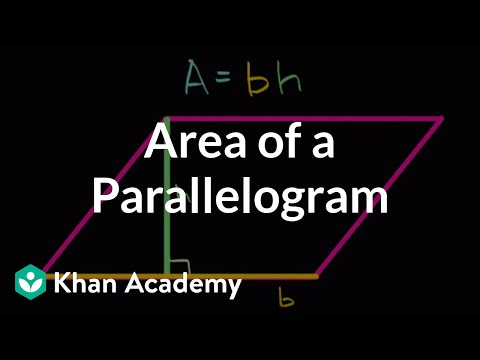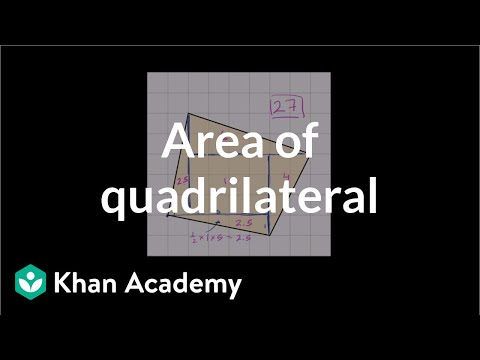Video

# Area of a triangle on a grid (Full video)

Description: Learn how to calculate the area of a triangle on a grid. Created by Sal Khan. What I would like to do is find the area of this green triangle. So whenever you start thinking about areas of triangle, or least my brain says, well look, I can figure out the area of a triangle if I know the base and the height of the triangle. So, for example, if I have a triangle that looks like this.

### Other videos you might be interested in### Area of a parallelogram (Full video)